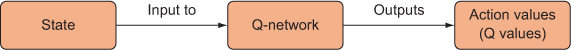# Chapter 4. Learning to pick the best policy: Policy gradient methods

### This chapter covers

• Implementing the policy function as a neural network
• Introducing the OpenAI Gym API
• Applying the REINFORCE algorithm on the OpenAI CartPole problem

In the previous chapter we discussed deep Q-networks, an off-policy algorithm that approximates the Q function with a neural network. The output of the Q-network was Q values corresponding to each action for a given state (figure 4.1); recall that the Q value is the expected (weighted average) of rewards.

##### Figure 4.1. A Q-network takes a state and returns Q values (action values) for each action. We can use those action values to decide which actions to take.Given these predicted Q values from the Q-network, we can use some strategy to select actions to perform. The strategy we employed in the last chapter was the epsilon-greedy approach, where we selected an action at random with probability ε, and with probability 1 – ε we selected the action associated with the highest Q value (the action the Q-network predicts is the best, given its experience so far). There are any number of other policies we could have followed, such as using a softmax layer on the Q values.